# PHYS 150 :: Physics Illinois :: University of Illinois at Urbana-Champaign

## Core Math

### Scientific notation

This course will take us from the scale of atoms to that of the universe. Along the way we'll encounter many numbers that are much larger are smaller than those from everyday experience. When dealing with very large or very small numbers, it is much more convenient (and takes a lot less typing) to use scientific notation. Scientific notation takes the form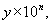and the rule is quite simple:

• If n is positive, move the decimal point n places to the right.
• If n is negative, move the decimal point n places to the left.
When typing, we often abbreviate scientific notation with an "e" instead of the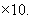. Many calculators also use this notation. Some examples: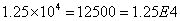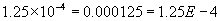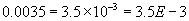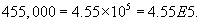### Algebra

We will use the following notation: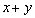means "x plus y"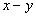means "x minus y"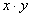means "x times y"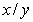means "x divided by y"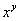means "x to the y power" or "x times itself y times"

Example: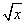means "the square root of x" and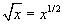### Geometry and Trigonometry

The figure shows a right triangle. It is a right triangle because one of the angles is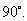The other two angles are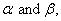and the sides have length a, b, and c.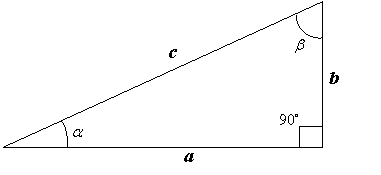We have the following relationships: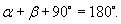Pythagorean Theorem: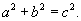Sine is opposite over hypotenuse: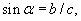and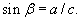Cosine is adjacent over hypotenuse: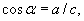and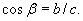Tangent is opposite over adjacent: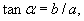and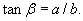### Prefixes

When discussing big or small numbers we'll sometimes use SI prefixes on units of measurement. Common examples include "kilometer" (instead of 103 meters), "milligram" (instead of 10-3 grams), "gigawatt" (instead of 109 watts), etc. I've listed them all below - we'll only need the first few rows!

 0.001 milli- (m) 1000 kilo- (k) 1e-06 micro- (μ) 1e+06 mega- (M) 1e-09 nano- (n) 1e+09 giga- (G) 1e-12 pico- (p) 1e+12 tera- (T) 1e-15 femto- (f) 1e+15 peta- (P) 1e-18 atto- (a) 1e+18 exa- (E) 1e-21 zepto- (z) 1e+21 zetta- (Z) 1e-24 yocto- (y) 1e+24 yotta- (Y)